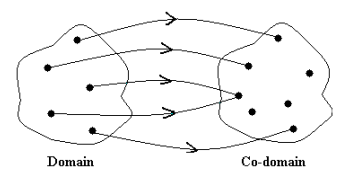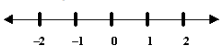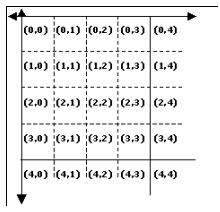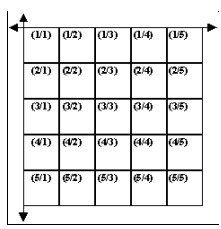Courses

# Description of Signals Electrical Engineering (EE) Notes | EduRev

## Electrical Engineering (EE): Description of Signals Electrical Engineering (EE) Notes | EduRev

The document Description of Signals Electrical Engineering (EE) Notes | EduRev is a part of the Electrical Engineering (EE) Course Signals and Systems.
All you need of Electrical Engineering (EE) at this link: Electrical Engineering (EE)

Description of Signals

What is a signal?
A signal, as stated before is a function of one or more independent variables. Recall that a function defines a correspondence between 2 sets, i.e.: corresponding to each element of one set (called the domain ), there exists a unique element of another set (called the codomain ) .Notice that more than one element in the domain may correspond to the same element in the co-domain .

A function is also sometimes referred to as a mapping. Thus a signal may also be defined as a mapping from one set to another. For example a speech signal would be mathematically represented by acoustic pressure as a function of time. Some more examples of signals are voltage, current or power as functions of time. A monochromatic picture can be described as a signal which is mathematically represented by brightness as a function of two spatial variables.

As mentioned earlier, there may be more than one independent variable. For example, the independent variable for a photograph is 2- dimensional space (2 space variables). The variables may also be hybrid, say 2 space variables and 1 time variable (E.g.: a video signal).

Note: In this course, we shall focus our attention on signals of only one variable. Also, for convenience, we shall generally refer to the independent variable as time. So don't let the recurring reference to time confuse you. It is symbolic for any independent variable you care to choose.

Discrete-time signals

Discrete variables are those in which there exists a neighbourhood around each value in which no other value is present.

ntuitively, it means a variable like the natural numbers on the real line - we can isolate each instance of the discrete variable from the other instances.

Why should we bother about discrete variables?

Discrete variables come up intrinsically in several applications. Take for example, the cost of gold in the market every day. The dependent variable (cost) is a function of discrete time (incremented once every day). Another example is the marks scored by the students in class. Here the dependent variable (marks) is a function of the discrete variable roll number. While it is perfectly fine to talk about marks of 02007005, it makes no sense to talk of marks of roll no 02007011.67 - this system is inherently discrete. Another point that should be noted here is that some results about signals and systems are common to both: continuous as well as discrete signals, but can be grasped more intuitively in one case as compared to the other. So, we shall pursue the study of both these cases simultaneously in this course.

Need the discrete variable be uniform?

No. though we imagine natural number or integers when we think of discrete signals, the points need not be equally spaced. For example, if the markets remained closed on Sundays, we would not record a price for gold on that day - so the spacing between the variables on this axis changes. In most common cases, however, the independent variable is uniform - and throughout this course, we shall assume a uniform spacing of the variable unless otherwise stated explicitly. This assumption makes the analysis more intuitive and also yields several good theorems for our use, which we shall see as we proceed.

Discrete-time signals

Discrete variables are those in which there exists a neighborhood around each value in which no other value is present.

Intuitively, it means a variable like the natural numbers on the real line - we can isolate each instance of the discrete variable from the other instances.

Why should we bother about discrete variables?

Discrete variables come up intrinsically in several applications. Take for example, the cost of gold in the market every day. The dependent variable (cost) is a function of discrete time (incremented once every day). Another example is the marks scored by the students in class. Here the dependent variable (marks) is a function of the discrete variable roll number. While it is perfectly fine to talk about marks of 02007005, it makes no sense to talk of marks of roll no 02007011.67 - this system is inherently discrete. Another point that should be noted here is that some results about signals and systems are common to both: continuous as well as discrete signals, but can be grasped more intuitively in one case as compared to the other. So, we shall pursue the study of both these cases simultaneously in this course.

Need the discrete variable be uniform?

No. though we imagine natural number or integers when we think of discrete signals, the points need not be equally spaced. For example, if the markets remained closed on Sundays, we would not record a price for gold on that day - so the spacing between the variables on this axis changes. In most common cases, however, the independent variable is uniform - and throughout this course, we shall assume a uniform spacing of the variable unless otherwise stated explicitly. This assumption makes the analysis more intuitive and also yields several good theorems for our use, which we shall see as we proceed.

Do discrete signals necessarily come from continuous signals? Although intuition may suggest so, this is not necessarily the case. In one of the example above - we considered the daily rate of gold. Here, time is intrinsically a continuous variable, and we made a discrete variable by taking measurements after certain intervals. However, the marks as a function of roll numbers intrinsically form a discrete system - there is no continuous axis of roll numbers.

Then how do we define the neighbourhood?

Okay, by now it may seem that we are hiding some details here - we defined a discrete variable as one in which no other value exists in a certain neighbourhood of one. Now for roll numbers, a neighbourhood does not make sense. How do we formally define a discrete signal?

A discrete variable is one which can ultimately be indexed by integers.

Examples of discrete variables

Now that we seem to have an intuitive understanding of what a discrete variable is, let us take some examples of discrete variables: First, the simplest and most intuitive discrete set is the integer axis itself:Then we can consider a set of tuples (a,b) such that a and b are both in the range 0 to 5 - how can we index them by integers?Now lets come to something that is discrete alright, but not very intuitive about how we can index it - rational numbers:We represent the rational numbers along the fourth quadrant, as y/x. The repeated areas (like 2/2, 3/3, 4/2 etc) are to be neglected, hence are in gray. Then we go on indexing them diagonally as shown by the animation. Now, we go ahead another step - how do we index a full plane?Note the method: we start in expanding circles from the origin. As soon as a circle cuts integer points, we pause and number the points clockwise from the positive y axis. This method is by no means unique - but just one set of indexing is enough for us to call the system “discrete”. Here we pause to note that although variables like the integer plane above can be indexed by integers, it is far more convenient to use tuples of integers to index them. It can mathematically be proved that any finite set of integers {a1 , a2 , a3 .... an } can be indexed by a single variable.

Representation of discrete variables

Let us decide some conventions for use with discrete variables:

• We shall mostly deal with time as the discrete variable, and shall denote it by “n” and keep “t” for continuous time.
• We will enclose discrete variables in brackets [.] as opposed to parenthesis (.) for continuous variables.
• A discrete signal is also called a sequence - the word coming from the familiar usage in mathematics.

Conclusion:

In this lecture you have learnt:

• Thus a signal may also be defined as a mapping from one set (domain) to another (co domain).
• Continuous-time signal means the mapping is defined over a continuum of values of the independent variable.
• A discrete variable is one which can ultimately be indexed by integers (may also be in terms of tuples) .
• We will enclose discrete variables in brackets [.] as opposed to parenthesis (.) for continuous variables.
The document Description of Signals Electrical Engineering (EE) Notes | EduRev is a part of the Electrical Engineering (EE) Course Signals and Systems.
All you need of Electrical Engineering (EE) at this link: Electrical Engineering (EE)Use Code STAYHOME200 and get INR 200 additional OFF Use Coupon Code
All Tests, Videos & Notes of Electrical Engineering (EE): Electrical Engineering (EE)

### Top Courses for Electrical Engineering (EE)## Signals and Systems

32 videos|74 docs|56 tests

### Top Courses for Electrical Engineering (EE)Track your progress, build streaks, highlight & save important lessons and more!

,

,

,

,

,

,

,

,

,

,

,

,

,

,

,

,

,

,

,

,

,

;